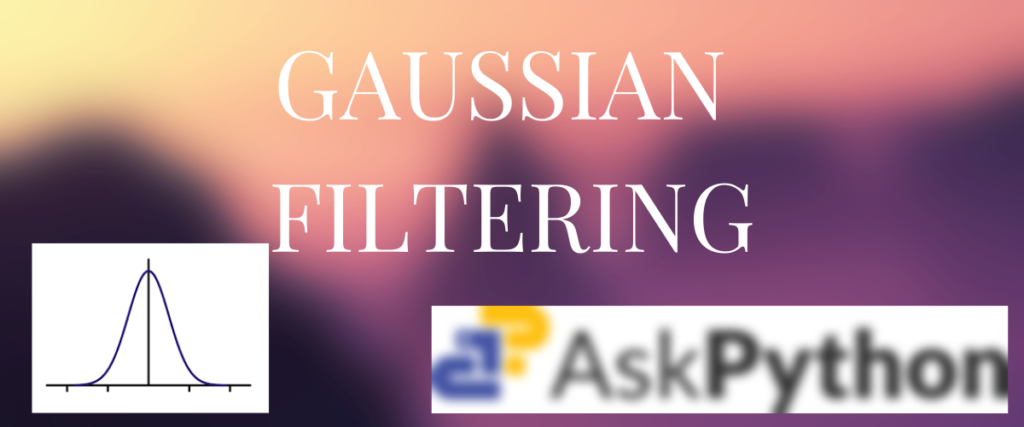# 3 Effective Methods for Applying Gaussian Filters to ImagesImage processing is a crucial technique for extracting information and enhancing image quality. The main tasks include image acquisition, restoration, enhancement, and segmentation. When we are talking about restoration and enhancement, there is one method we commonly use to remove the noise in the image and enhance the quality of the image which is filtering.

Applying Gaussian filters to images effectively reduces noise and enhances quality. This article outlines three approaches to Gaussian filtering: using MATLAB’s `imgaussfilt`, applying Scipy’s `gaussian_filter`, and leveraging OpenCV’s `GaussianBlur`. Each method relies on the standard deviation (sigma) to control the blur intensity. The Gaussian kernel, based on the Gaussian distribution, offers a bell-shaped curve for more natural blurring compared to box kernels.

## Understanding the Gaussian Kernel: More Than Just a Filter

In image processing, a kernel also called a filter or a convolution matrix is used to carry out blurring, sharpening, edge detection, and other related tasks.

Most of the filtering methods you see use a box filter(known as a mean filter). However, the Gaussian kernel is based on the Gaussian distribution, popularly known as the normal distribution. The important characteristic? This kernel is bell-shaped.

The noise in the image is removed according to the kernel size. If the kernel size is increased, the blur intensity is increased. The Gaussian Kernel is also significant in Support Vector Machines (SVM).

The Gaussian Filter is the operation of using the kernel to blur the images by applying the kernel over a matrix of pixels.

## How to Apply Gaussian Filters Using MATLAB

Let us understand how we can apply a Gaussian filter on images using MATLAB.

MATLAB has its very own method called `imgaussfilt` for applying the Gaussian blur. The Gaussian filter relies on a parameter known as the standard deviation, commonly referred to as sigma. The standard deviation we use for Gaussian filtering is 1. Sigma is an important parameter even in the approaches we are going to see later.

```img = imread("<File path>");
sigma = 1;
filtered_img = imgaussfilt(img, sigma);
figure;
subplot(1, 2, 1);
imshow(img);
title('Original Image');
subplot(1, 2, 2);
imshow(filtered_img);
title('Gaussian Filtered Image');
```

In the very first line, we are reading the image into `img`. Remember to replace the <file path> with the actual file path of the image.

Next, we are defining a new variable called sigma to store the standard deviation value. The `imggaussfilt` method is used to apply the Gaussian Filter to the original image. Lastly, we are displaying the original image and the filtered image in a single grid. The titles are also displayed for both images.

The original image has some static noise which is removed when the image is blurred.

In this example, the standard deviation, also known as sigma, is set to 1. If we like to see an even more blurry image, we’d have to increase the sigma value.

## Using Scipy to Apply Gaussian Filters

The scipy library can also be used to apply blurring to the image with the help of its very own method – the gaussian_filter.

```import matplotlib.pyplot as plt
import imageio.v2 as imageio
from scipy.ndimage import gaussian_filter
sigma = 1
filtered_img = gaussian_filter(img, sigma=sigma, order=0)
plt.subplot(1, 2, 1)
plt.imshow(img)
plt.title('Original Image')
plt.subplot(1, 2, 2)
plt.imshow(filtered_img)
plt.title('Gaussian Filtered Image')
plt.show()
```

In the first three lines, we import the matplotlib library for plotting the images. Then imageio is used to read the images. And finally, gaussian_filter method of the scipy library is used to apply the Gaussian Filter on the image.

We are reading the original image using imageio and storing it in a new variable called img. We are also defining the standard deviation value equal to 1. The Gaussian filter method is used to blur the image. Lastly, we are displaying the original and blurred images.

## Applying Gaussian Filters with OpenCV: A Practical Guide

OpenCV is the most sought tech stack for dealing with and manipulating images. It has many functions for any image-related tasks you can probably think of.

Thanks to OpenCV, we can apply Gaussian Filter with the help of `GaussianBlur`.

```import cv2
import matplotlib.pyplot as plt
img = '/content/gfilter4.jpeg'
if src is not None:
dst = cv2.GaussianBlur(src, (5, 5), cv2.BORDER_DEFAULT)
fig, axes = plt.subplots(1, 2, figsize=(10, 5))
axes.imshow(src)
axes.set_title('Original Image')
axes.imshow(dst)
axes.set_title('Gaussian Filtered Image')
for ax in axes:
ax.axis('off')
plt.show()
else:
print(f"Error: Could not read the image from {img}")
```

In the first two lines, we import the OpenCV(cv2) and matplotlib libraries. The path to the image is stored in a variable called image and is read using the `imread` method into a new variable called src. We are writing an if condition to check if the image is read successfully. Only then, we can carry out the filtering process. If the image is not read successfully, an error is displayed according to the last line. Inside the loop, we are applying the GaussianBlur method with a kernel size of 5. The result of this operation is saved in dst. The matplotlib library is used to display the images as subplots.